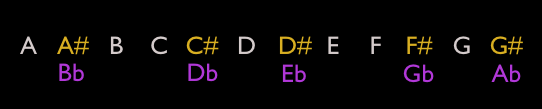﻿ Basics of the Music System | Cosmometry

# Basics of the Music System

12-Tone and 7-Tone Scales

The 12-tone musical scale consists of — you guessed it — 12 tones, or notes, labeled alphabetically as:This is called the “chromatic” scale — 12 equal-interval steps up the scale (called “half steps”). As you can see, there are seven letters (A B C D E F G) and five sharps and flats (i.e. A#/Bb). The seven letters comprise the 7-note “diatonic scale” we’re all familiar with as “do, re, mi, fa, sol, la, ti…do.” In the key of C, these are C D E F G A B…C (the ending C is the beginning of the next “octave” wherein the pattern repeats at double the pitch (frequency) of the previous scale). This 7-tone scale consists of a combination of whole steps (two-note intervals) and half steps (one-note intervals) along the 12-tone chromatic scale. This 7-tone pattern is the same in all twelve keys.

Tri-Tones

Music is a system of relationships, these being the harmonic intervals between notes and the combination of these intervals to create melodies and harmonies. One of the primary interval relationships is called the tri-tone due to it being an interval of two notes that are 3 whole steps apart. Given that 3 whole steps is equal to 6 half steps, we find that these two notes exactly divide the 12-tone scale in half. As such, they can be seen as two primary “opposite” notes. In fact, the tri-tone interval, when sung or played on an instrument, is perhaps the most unresolved harmonic relationship in all of music. It is full of tension and dissonance, and yet is it present in almost every piece of music composed.

Being that the tri-tone consists of two opposite notes in the 12-tone system, it’s obvious then that there would be six pairs of these tri-tone intervals. These are colored coded in the diagram below as A/D#, A#/E, B/F, C/F#, C#/G, D/G#.Note for later that the color coding is intentionally spectral as such:  A, B, C#, D, E, F#, G# . These are the seven notes of the major scale in the key of A.

Circle of Fifths

Another very basic harmonic relationship is called the fifth. Simply, it’s the interval between the first and fifth notes of a major scale. For example, in the key of A the fifth note is E (A=1, B=2, C#=3, D=4, E=5, etc). This interval is so primary and profound in music it is actually called the “perfect fifth.” In the world of classical music theory, one of the ways to show fundamental relationships is to array the 12 notes around a circle following a sequence of fifths, as in the illustration below. This shows that there is a progression in music that naturally moves or cycles through all 12 keys in a way that is harmonically pleasing to our senses (it is also referred to as the cycle of fifths).

Using the 12-around-1 matrix pattern as our guide, the circle of 5ths looks like this (with the spectral color-coding as mentioned above):

And this illustration shows that the tri-tone intervals are exactly opposite each other in the circle of fifths:

The same holds true when the 12-tone system is arrayed chromatically around the circle.

In this way we can easily see the six pairs of opposite notes that comprise the basic 12-tone system of music.

Extending this into the 3-dimensional space of the vector equilibrium, one of the primary components of cosmometry, we can map these pairs like this (notice that the circle of fifths is maintained in the sequence of notes as they are arrayed visually around the center):

Buckminster Fuller stated that the vector equilibrium is composed of six-pairs of equal and opposite vectors radiating from its center point. The music system aligns with that model conceptually, though of course the frequency differences of the actual tri-tone notes are not equal.

Basic Interval Polygons

With the interconnecting lines of the 12-around-1 matrix as our guide, we can then see that there is a set of basic interval relationships that map out polygonal patterns corresponding to specific intervals. As seen below, these are:

•  One 12-sided dodecagon ringing the outside… our circle of fifths

•  Two 6-sided hexagons… showing two sets of whole-tone (whole step) scales

•  Three 4-sided squares… showing three sets of diminished (minor third) chords

•  Four 3-sided triangles… showing four sets of augmented (major third) chords

•  One 12-pointed dodecagram… showing the chromatic sequence of intervals

Six lines… showing our tri-tone interval pairs

Note that the color-coding of these polygons is not coordinated with the above color-coding but is simply to make them easier to see in this illustration.

Next... we'll explore a fundamental aspect of the music system in The Tri-Tone Duality of Music.